CBSE Class 12 Sample Paper for 2023 Boards

Class 12
Solutions of Sample Papers and Past Year Papers - for Class 12 Boards

## (d) A is false but R is true.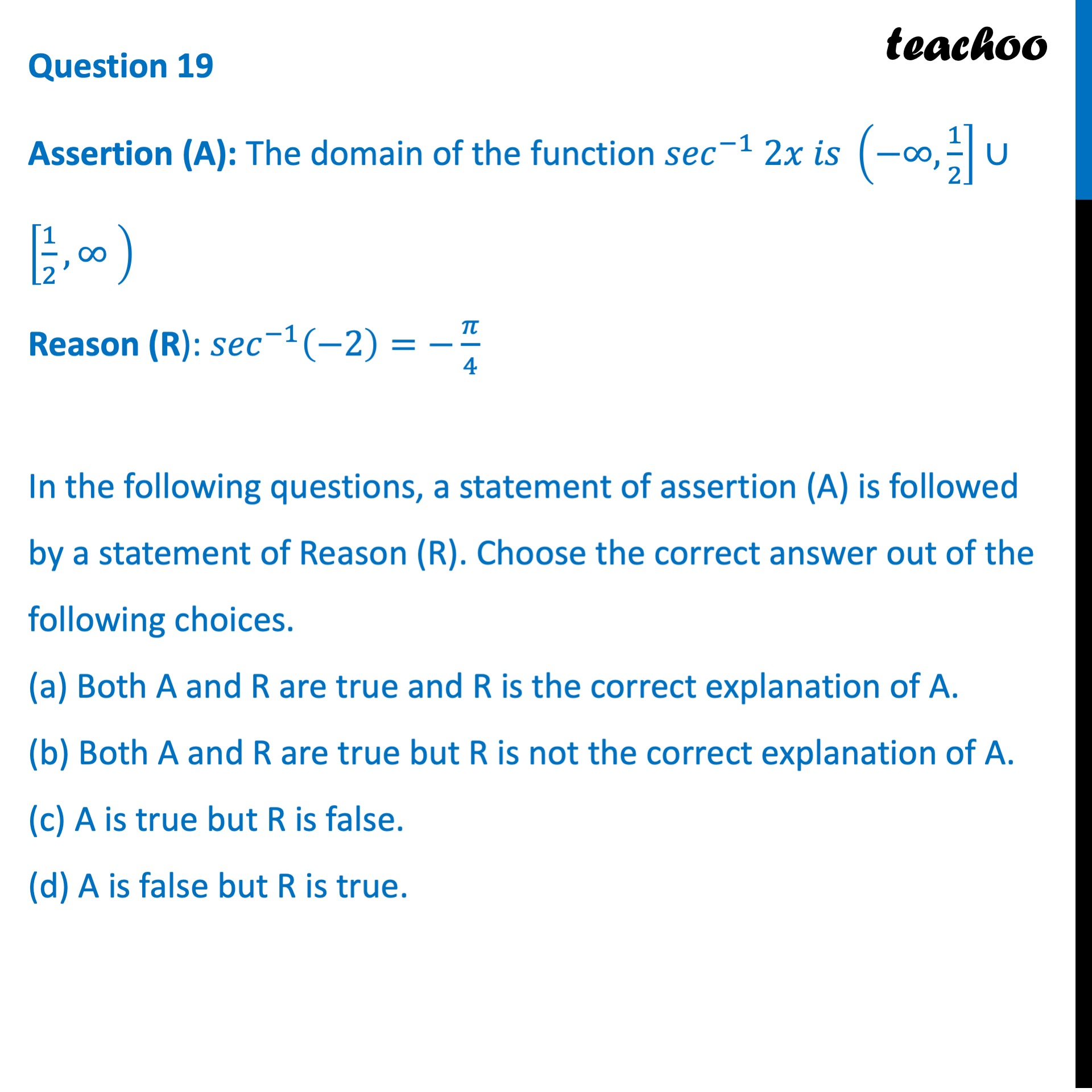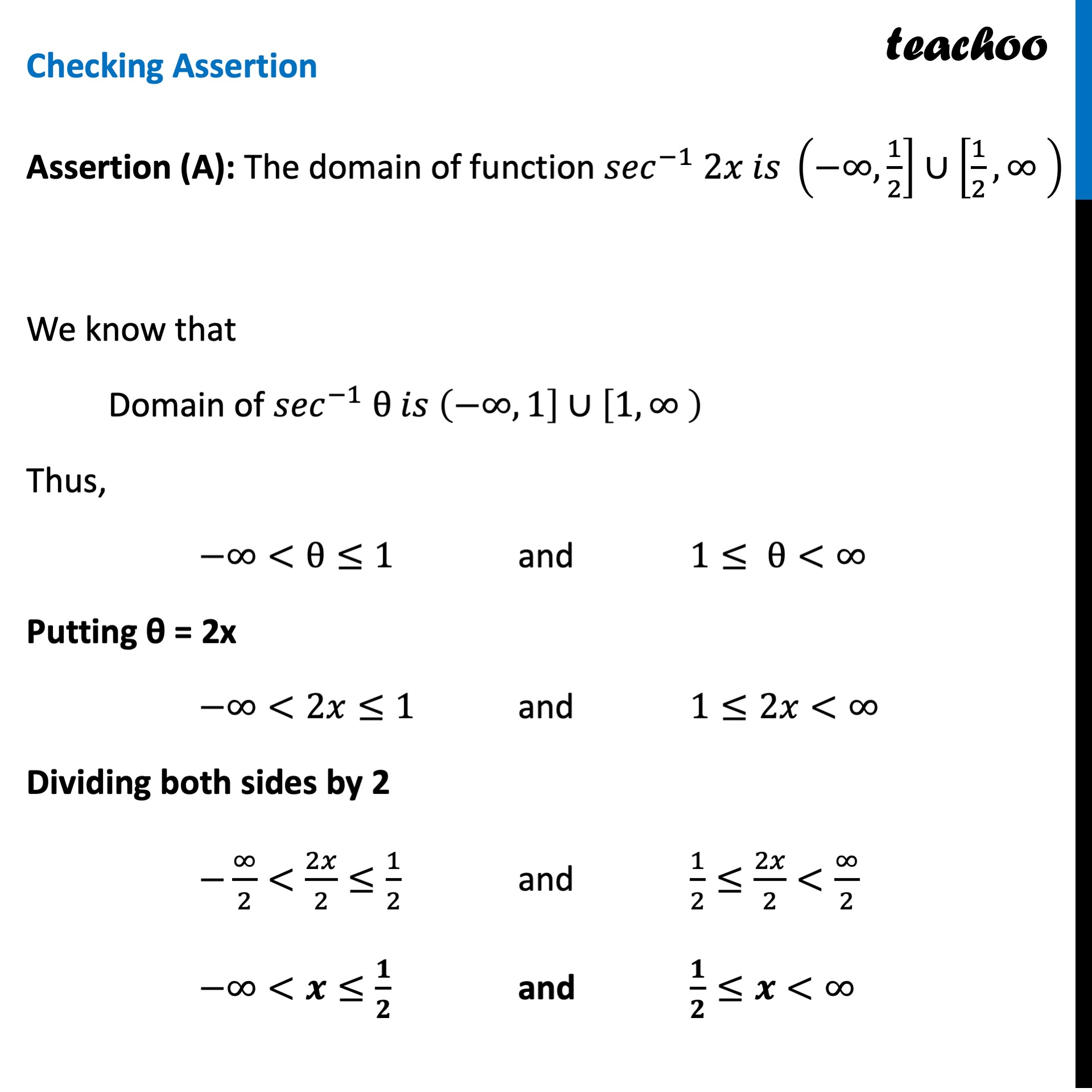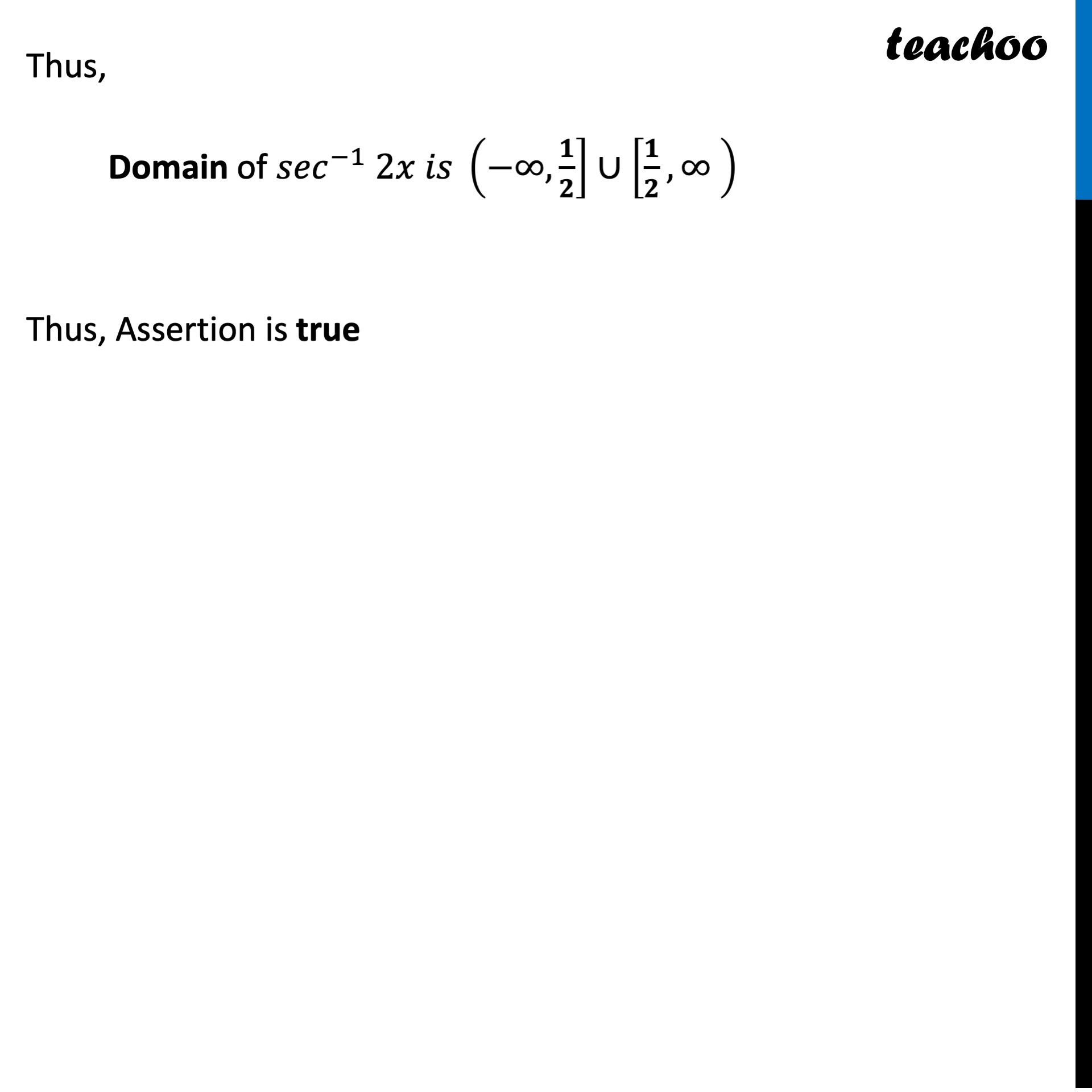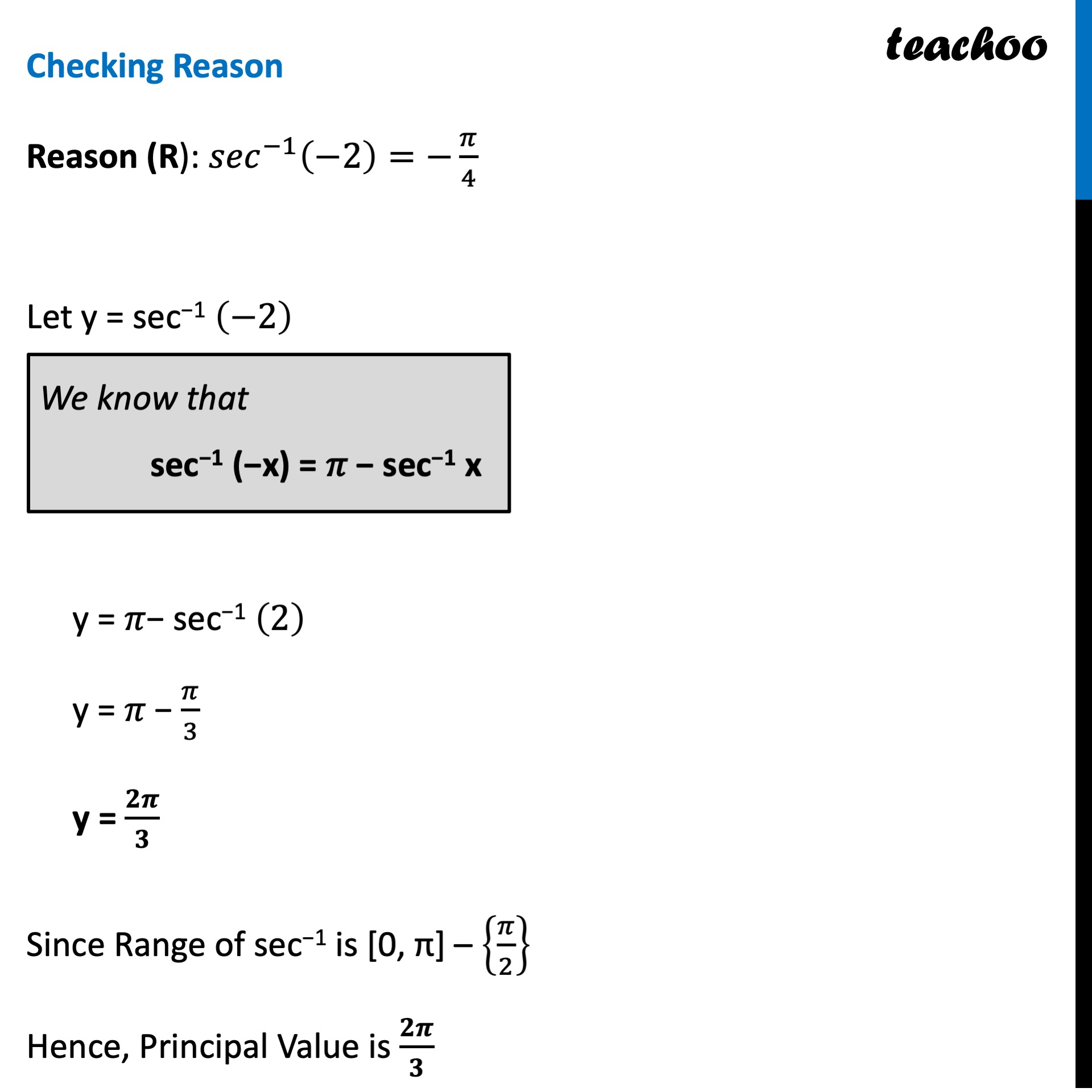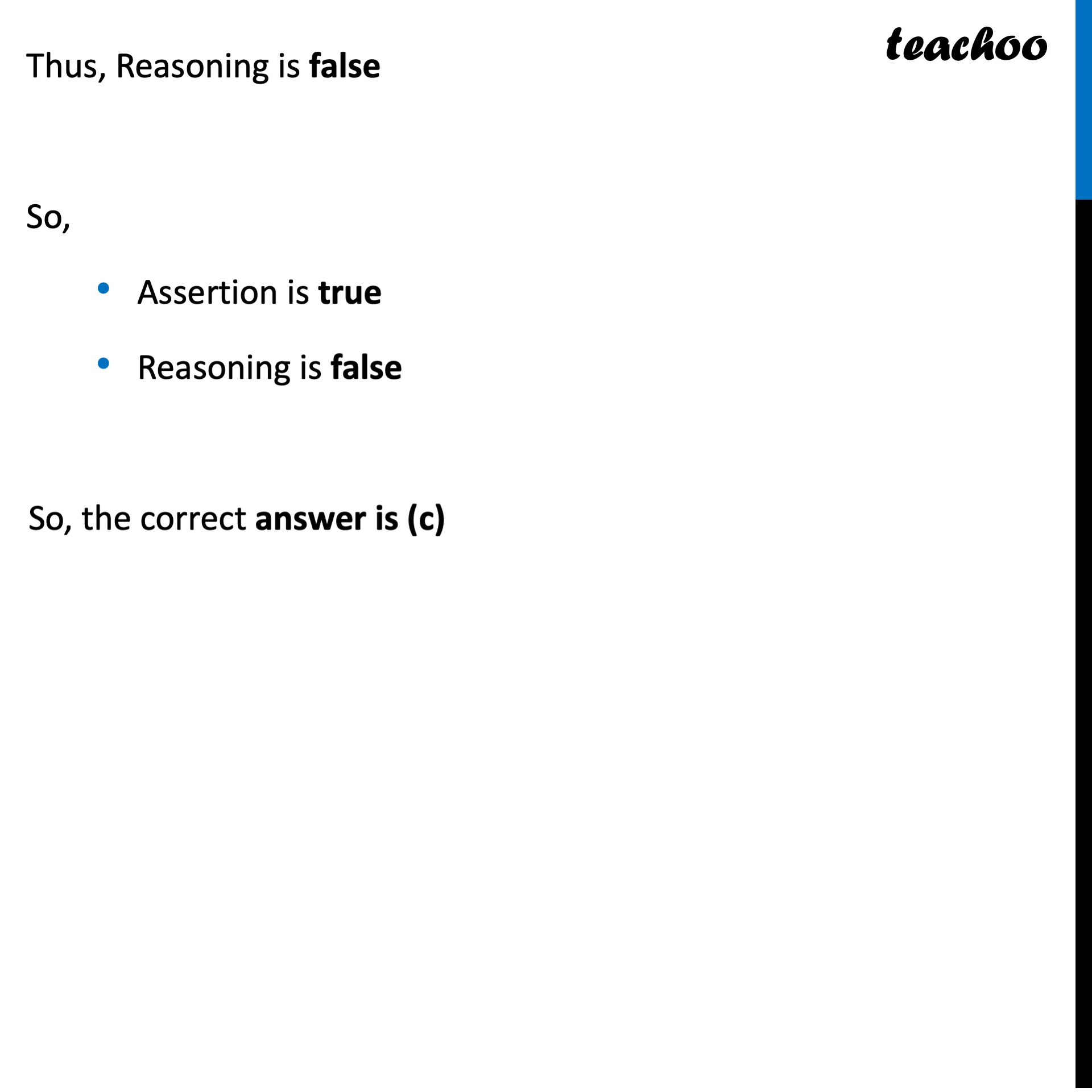Learn in your speed, with individual attention - Teachoo Maths 1-on-1 Class

### Transcript

Question 19 Assertion (A): The domain of the function 〖𝑠𝑒𝑐〗^(−1) 2𝑥 𝑖𝑠 (−∞,1/2]∪[1/2,∞ ) Reason (R): 〖𝑠𝑒𝑐〗^(−1) (−2)=−𝜋/4 In the following questions, a statement of assertion (A) is followed by a statement of Reason (R). Choose the correct answer out of the following choices. (a) Both A and R are true and R is the correct explanation of A. (b) Both A and R are true but R is not the correct explanation of A. (c) A is true but R is false. (d) A is false but R is true. Checking Assertion Assertion (A): The domain of function 〖𝑠𝑒𝑐〗^(−1) 2𝑥 𝑖𝑠 (−∞,1/2]∪[1/2,∞ ) We know that Domain of 〖𝑠𝑒𝑐〗^(−1) θ 𝑖𝑠 (−∞,1]∪[1,∞ ) Thus, −∞<θ≤1 and 1≤ θ<∞ Putting θ = 2x −∞<2𝑥≤1 and 1≤2𝑥<∞ Dividing both sides by 2 −∞/2<2𝑥/2≤1/2 and 1/2≤2𝑥/2<∞/2 −∞<𝒙≤𝟏/𝟐 and 𝟏/𝟐≤𝒙<∞ Thus, Domain of 〖𝑠𝑒𝑐〗^(−1) 2𝑥 𝑖𝑠 (−∞,𝟏/𝟐]∪[𝟏/𝟐,∞ ) Thus, Assertion is true Checking Reason Reason (R): 〖𝑠𝑒𝑐〗^(−1) (−2)=−𝜋/4 Let y = sec−1 (−2) y = 𝜋− sec−1 (2) y = 𝜋 − 𝜋/3 y = 𝟐𝝅/𝟑 Since Range of sec−1 is [0, π] – {𝜋/2} Hence, Principal Value is 𝟐𝝅/𝟑 Thus, Reasoning is false So, Assertion is true Reasoning is false So, the correct answer is (c)